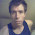## Wednesday, February 13, 2008

### Metric spaces, VC-dimension, and metric entropy.

For a problem that I've been working on, it turns out that if a related range space has bounded VC-dimension, the problem can be solved exactly (but with running time exponential in the dimension). The range space is constructed from two parameters: a metric space (X, d), and a radius e, and consists of the domain X, and all balls of radius at most e in X.

So a natural question that I've been unable to answer is:
What properties of a metric space ensure that the induced range space has bounded VC dimension ?
Most of what we do know comes from the PAC-learning community. For instance, the doubling dimension of a metric space is the smallest number d such that any ball of radius e can be covered by at most 2^d balls of radius e/2. In recent years, it has been popular to explore the extent to which
"metric space of bounded doubling dimension == bounded dimensional Euclidean space"
is true. Unfortunately, there are spaces of bounded VC-dimension that do not have bounded doubling dimension.

Another related proxy for the "dimension" of a metric space is its metric entropy: Determine the minimum number of balls of radius e needed to cover all points in a metric space. The log of this number is the metric entropy, and among other things is useful as a measure of the number of points needed to "cover" a space approximately (in a dominating set sense). It's known that the metric entropy of concept classes is closely related to their VC-dimension, (where the underlying metric is symmetric difference between the classes). I am not aware of any general result that relates the two though.

For more on the dizzying array of numbers used to describe metric space dimension, do read Ken Clarkson's magnificent survey.

[On a side note, I don't quite understand why the term "entropy" is used. It seems to me that if one wanted to use entropy, one would compute the entropy of the resulting set of balls, rather than merely the log of their number. ]

1.I had always thought that "metric entropy" was by analogy to the Boltzmann entropy in statistical mechanics, which is the log of the number of microstates compatible with a given macroscopic state. (This actually predates the Gibbs-Shannon entropy.)

2.This is a very interesting question. In the random graph, with distance understood as graph distance, the balls of radius e would have infinite VC dimension for every e. On the other hand, for any metric definable in an o-minimal structure, the balls of radius e would have finite VC dimension for every e. You could go further and say that in any metric structure which is NIP (not the independence property) the balls of radius e will have finite VC dimension for every e. Unfortunately this is a bit tautologous, because NIP structures are precisely those in which all formulas have finite VC dimension.

The challenge seems to be to relate the NIP condition to an ordinary classification characteristic of metric spaces. Looking at the wikipedia entry for Metric Space I see no promising properties. The usual labels of Separable, Connected, Compact, etc seem to be irrelevant.

Actually, I notice that in the random graph, all points are distance 0,1, or 2 apart. This may be relevant.

It is worth noting that this question is equivalent to the question: For which metric spaces does the set of e balls have finite independence dimension?

A simpler question to consider asking first is: what graphs, with distance understood as graph distance, have this property?

In mathematical logic the idea of NIP is taken somewhat as a primitive. It is not known to be reducible to other seemingly orthogonal properties. One might consider reading Hans Adler's nice summary of what is known about equivalent conditions for NIP.

A promising hunting ground seems to be new work in continuous logic, which is inherently metric. See the following for an overview: http://www.aimath.org/~skrantz/Blurbs/model.html

3.thanks ! that's very interesting...